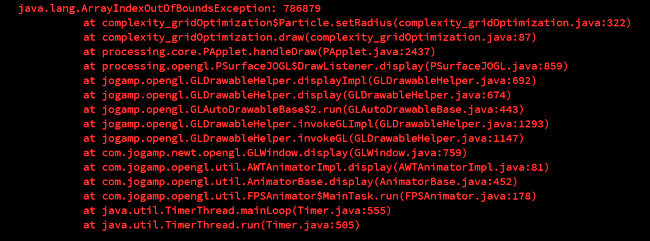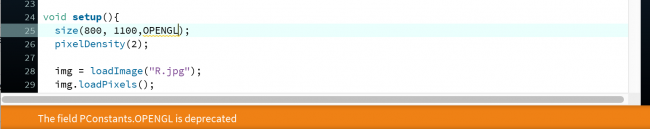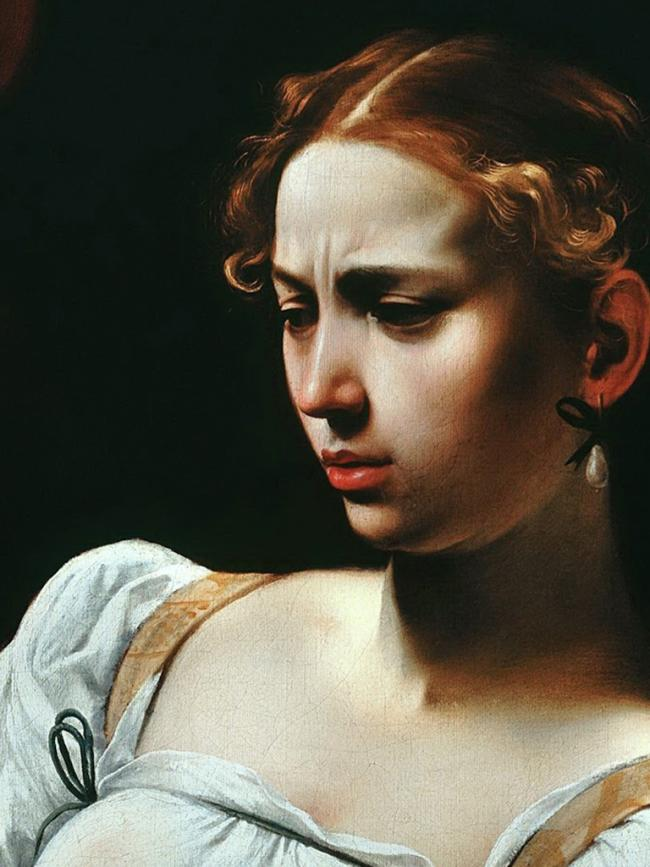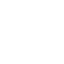#### Howdy, Stranger!

We are about to switch to a new forum software. Until then we have removed the registration on this forum.

# OpenGL (The field PConstants.OPENGL is deprecated)

edited February 2018

Hi everyone;

The code I'm working on is being stopped after a while, can you help me on this thing?

Error warning: "The field PConstants.OPENGL is deprecated"

Tagged:

• In size()? Use P3D or P2D

• How could be check use which one ? Cuz ı'm new user Processing.

• It would help us a lot if you were to POST THE CODE that is giving you this error..!

• Is the project in 2d or 3d?

If you don't know then try them both, one at a time.

But, yes, hard to know what you're asking without code.

• Error code;

`ArrayIndexOutOfBoundsException 805454`

• P2D is 2D OpenGL. P3D is 3D OpenGL.

Are you making a 2D or 3D sketch?

For details, just look the line giving you the error up in the reference! For size, see:

It explains what constants you can use, and what each one means.

• Make 2D sketch, usually stop working.

•Like that Error, really need help.

• just write size(800,1100); without any third parameter

Chrisir

• That error is nothing to do with opengl. (I'm guessing you're accessing pixels outside of the image you're losing)

Post your code. Don't post pictures of your code. Format it nicely.

• really need help.

Then post your code. We are guessing otherwise. We asked yesterday. We could've fixed it by now.

• ``````import java.util.*;
import java.awt.Rectangle;

PImage sprite;

ArrayList<Particle> particles;
ArrayList<Particle> check;
Particle newp;
Grid qt;
PGraphics circle;

int nParticles = 15000;
int count = 0;
PImage img;
float rythm = 0;
float growRatio = 0.8;
Particle p;
float g = 50.0;
boolean debugMode = false;
int debugId = 0;
float distance = 2;
float angle = random(TWO_PI);

void setup(){
size(1200, 700,OPENGL);
pixelDensity(2);

qt = new Grid(48,28);
particles = new ArrayList<Particle>();
check = new ArrayList<Particle>();
for (int i=0; i<1; i++){
count++;
}
noStroke();
fill(255);
hint(DISABLE_DEPTH_TEST);
//hint(ENABLE_ACCURATE_2D);
}

void draw(){

background(0);
fill(255);

qt.clear();

for (int i = 0; i < particles.size(); i++) {
qt.insert(particles.get(i));

}
for (int i = particles.size()-1; i >= 0; i--) {
p = particles.get(i);
check = qt.getInsideCircle(p,30);
for (int j = 0; j < check.size(); j++){
if (p.id != check.get(j).id){
p.applyForce(check.get(j).attract(p));
}
}

p.update();
drawParticle(p);
//p.display();

if (p.age > p.spawnAge && p.velocity.magSq() < 1 && !p.spawned && count<nParticles){
p.spawned = true;
angle = random(TWO_PI);
//angle -= PI;

newp = new Particle(p.location.x+sin(angle)*distance,p.location.y+cos(angle)*distance,count);
count++;
newp.spawnAge+=floor(rythm);
newp = new Particle(p.location.x-sin(angle)*distance,p.location.y-cos(angle)*distance,count);
newp.spawnAge+=floor(rythm);
count++;
rythm+=growRatio;

}

}

println(frameRate);

if (debugMode){
text("count:" + count, 10, height-30);
p = particles.get(debugId);
fill(255,0,0);
p.display();
fill(0,255,0);
check = qt.getInsideCircle(p,30);
for (int j = 0; j < check.size(); j++){
if (p.id != check.get(j).id){
check.get(j).display();
}
}
stroke(50);
qt.drawGrid();
noStroke();
}

}

void drawParticle(Particle p) {
//noStroke();
tint(255);
texture(sprite);
normal(0, 0, 1);
vertex(p.location.x - p.r, p.location.y - p.r, 0, 0);
vertex(p.location.x + p.r, p.location.y - p.r, sprite.width , 0);
vertex(p.location.x + p.r, p.location.y + p.r, sprite.width, sprite.height);
vertex(p.location.x - p.r, p.location.y + p.r, 0, sprite.height);
endShape();
}

void keyPressed(){
if (key=='d'){
debugMode = !debugMode;
println(particles.get(debugId).id);
}
}
``````
• And try, without OPENGL doesnt work animation.

• Project made four parts, if i need other parts of course add here.

Thanks for help.

thank you

• and the images. we need the images.

• ``````class Grid{
ArrayList[] grid;
int cellWidth;
int cellHeight;

int rows,cols;

public Grid(int _cols, int _rows){
grid = new ArrayList[_rows*_cols];

rows = _rows;
cols = _cols;

cellWidth = width/cols;
cellHeight = height/rows;
}

void clear(){
for (int i = 0; i<grid.length; i++){
grid[i] = null;
}
}

int getParticlePosition(Particle p){
int xpos =  floor(p.location.x/cellWidth);
int ypos =  floor(p.location.y/cellHeight);
return (ypos*cols) + xpos;

}

void insert(Particle p){
int index = getParticlePosition(p);

if (grid[index] == null){
grid[index] = new ArrayList<Particle>();
}
}

ArrayList getCell(Particle p){
int index = getParticlePosition(p);
return  grid[index];
}

ArrayList getNear(Particle p){
ArrayList objects = new ArrayList<Particle>();

int xpos =  floor(p.location.x/cellWidth);
int ypos =  floor(p.location.y/cellHeight);

int xstart = constrain(xpos-1,0,cols);
int ystart = constrain(ypos-1,0,rows);
int xend = constrain(xpos+2,0,cols);
int yend = constrain(ypos+2,0,rows);

for (int x = xstart; x<xend; x++){
for (int y= ystart; y<yend; y++){
int index = x + y*cols;
}
}

return objects;
}

ArrayList<Particle> objects = getNear(p);
for (int i = objects.size()-1; i>=0; i--){
Particle tempP = objects.get(i);
PVector dist = PVector.sub(p.location,tempP.location);
objects.remove(i);
}
}

return objects;
}

void drawGrid(){
for (int i = 0; i < cols; i++){
line (cellWidth*i,0,cellWidth*i,height);
}
for (int i = 0; i < rows; i++){
line (0,i*cellHeight,width,i*cellHeight);

}
}

}
``````
• ``````class Particle {

PVector location;
PVector velocity;
PVector acceleration;
PVector force,friction;
float mass,r,distance,loc;
float maxForce, maxSpeed,strength;
int id,val;
color c;
float k = 0.02;
int age = 0;
int spawnAge = (int)random(80,120);
float minr = 0;
float maxr = 5.0;
boolean spawned = false;

Particle(float x, float y,int _id) {
mass = 2;
id = _id;
r = 2;
maxSpeed = 0.1;
maxForce = 50;
location = new PVector(constrain(x,r,width-r), constrain(y,r,height-r));
velocity = new PVector(0, 0);
acceleration = new PVector(0, 0);
}

void applyForce(PVector force) {
//PVector f = PVector.div(force, mass);
}

void update() {
velocity.limit(maxSpeed);
acceleration.mult(0);
friction();
borders();
age+=1;
}

void friction(){
friction = velocity.copy();
friction.mult(-1);
friction.normalize();
friction.mult(k);

applyForce(friction);
}

void display() {

ellipse(location.x,location.y,r*2,r*2);
}

PVector attract(Particle p) {
force = PVector.sub(p.location,location);             // Calculate direction of force
distance = force.magSq();

distance = constrain(distance, 1.0, 5000.0);                             // Limiting the distance to eliminate "extreme" results for very close or very far objects
force.normalize();                                            // Normalize vector (distance doesn't matter here, we just want this vector for direction

strength = (g) / (distance*4*PI); // Calculate gravitional force magnitude
force.mult(strength);     // Get force vector --> magnitude * direction
return force;

}

loc = (int)location.x + (int)location.y*img.width;
//c= img.get((int)location.x,(int)location.y);
//c =  img.pixels[int(loc)];
val = img.pixels[int(loc)] & 0xFF;
r=  lerp(r,map(val,0.0,255.0,minr,maxr),0.01);

}

void borders() {
if ( location.x < r || location.x > width-r){
velocity.x = -velocity.x;
}
if ( location.y < r || location.y > height-r){
velocity.y = -velocity.y;
}

if (location.x> width-r) location.x = width-r;
if (location.x< r) location.x = r;
if (location.y> height-r) location.y = height-r;
if (location.y< r) location.y = r;

}

}
``````
• ``````class Quadtree {
private int maxObjects = 10;
private int maxLevels = 5;

private int level;
private ArrayList<Particle> objects;
private Rectangle bounds;

level = pLevel;
objects = new ArrayList<Particle>();
bounds = pBounds;
}

void clear() {
if (objects.size() > 0){
objects.clear();
}
for (int i = 0; i < nodes.length; i++) {
if (nodes[i] != null) {
nodes[i].clear();
nodes[i] = null;
}
}
}

void split() {
int subWidth = (int)(bounds.getWidth() / 2);
int subHeight = (int)(bounds.getHeight() / 2);
int x = (int)bounds.getX();
int y = (int)bounds.getY();

nodes = new Quadtree(level+1, new Rectangle(x + subWidth, y, subWidth, subHeight));
nodes = new Quadtree(level+1, new Rectangle(x, y, subWidth, subHeight));
nodes = new Quadtree(level+1, new Rectangle(x, y + subHeight, subWidth, subHeight));
nodes = new Quadtree(level+1, new Rectangle(x + subWidth, y + subHeight, subWidth, subHeight));
}

private int getIndex(Particle pParticle) {
int index = -1;
double verticalMidpoint = bounds.getX() + (bounds.getWidth() / 2);
double horizontalMidpoint = bounds.getY() + (bounds.getHeight() / 2);

// Object can completely fit within the top quadrants
boolean topQuadrant = (pParticle.location.y + pParticle.r < horizontalMidpoint);
// Object can completely fit within the bottom quadrants
boolean bottomQuadrant = (pParticle.location.y - pParticle.r > horizontalMidpoint);

// Object can completely fit within the left quadrants
if (pParticle.location.x + pParticle.r < verticalMidpoint) {
index = 1;
}
index = 2;
}
}
// Object can completely fit within the right quadrants
else if (pParticle.location.x - pParticle.r > verticalMidpoint) {
index = 0;
}
index = 3;
}
}

return index;
}

/*
* Insert the object into the quadtree. If the node
* exceeds the capacity, it will split and add all
* objects to their corresponding nodes.
*/
void insert(Particle pParticle) {
if (nodes != null) {
int index = getIndex(pParticle);

if (index != -1) {
nodes[index].insert(pParticle);

return;
}
}

if (objects.size() > maxObjects && level < maxLevels) {
if (nodes == null) {
split();
}

int i = 0;
while (i < objects.size()) {
int index = getIndex((Particle)objects.get(i));
if (index != -1) {
nodes[index].insert((Particle)objects.remove(i));
}
else {
i++;
}
}
}
}

List retrieve(List returnObjects, Particle pParticle) {
int index = getIndex(pParticle);
if (nodes != null){
if (index != -1) {
nodes[index].retrieve(returnObjects, pParticle);
}else{
for (int i = 0; i <nodes.length;i++){
nodes[i].retrieve(returnObjects, pParticle);

}
}

}
return returnObjects;
}
}
``````
•Project does not progress with the same image and I have to change it from time to time.

• is that sprite or is that background3?

• background3, sprite is upload now and sprite image png and left bottom in comment.• that's working fine here. ran without errors for about 10 minutes

Processing 3.3, MacBook Pro (Retina, 15-inch, Mid 2015), AMD Radeon R9 M370X 2048 MB + Intel Iris Pro 1536 MB

• it doesn't work without the third argument to size() (because it's using textures)

but the following all work here

``````size(1200, 700, P2D);
size(1200, 700, P3D);
size(1200, 700, OPENGL);
``````
• Well, is the source of the error I sometimes experience with different measurements or visuals related to software or hardware?

My Hardware and Software Processing 3.3.6- MacbookPro( 13 Retina- early 2015) Intel Iris Graphics 6100